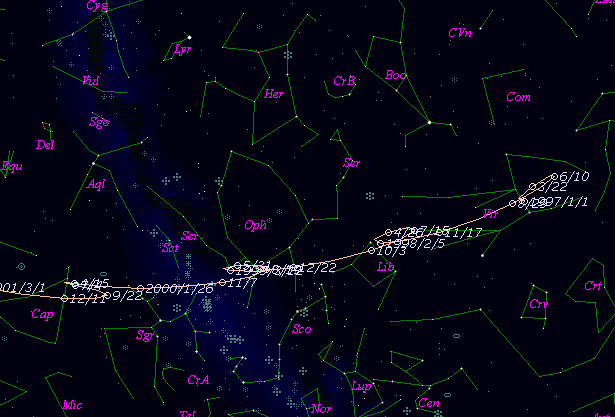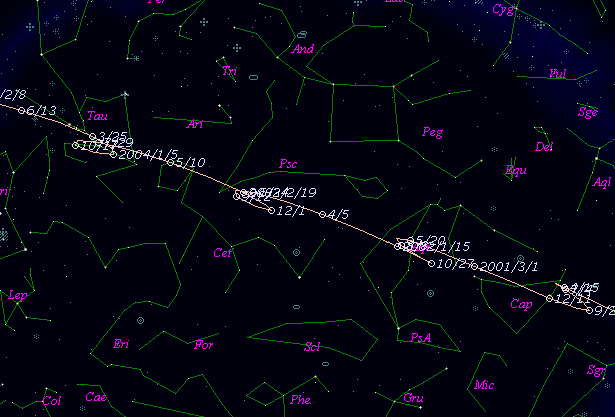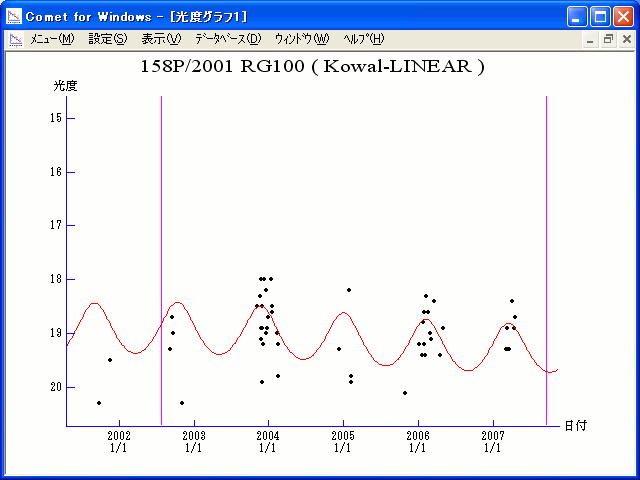# \$B%3%o%k(B-\$B%j%K%"WB@1(B

158P/Kowal-LINEAR (2002)###\$B%W%m%U%#!<%k(B

 \$BId9f(B 158P/2001 RG100 \$B:FH/8+F|(B 2001\$BG/(B9\$B7n(B12\$BF|(B \$B:FH/8+8wEY(B 19.1\$BEy(B \$B:FH/8+ Lincoln Laboratory Near-Earth Asteroid Research project

###\$B50F;MWAG(B

```Epoch 2002 July 25.0 TT = JDT 2452480.5
T 2002 July 25.61893 TT                                 MPC
q   4.5949872            (2000.0)            P               Q
n   0.09576196     Peri.  235.57304     +0.96950264     -0.22665878
a   4.7315927      Node   137.31333     +0.24444552     +0.92167460
e   0.0288709      Incl.    7.90313     -0.01763579     +0.31486779
P  10.29
```

###\$B@1?^(B###\$B8wEYJQ2=(B

```        m1 = 9.0 + 5 log\$B&\$(B + 10.0 log r
```##### \$B50F;MWAG\$O(BM.P.E.C. 2003-W74\$B\$K7G:\\$5\$l\$?\$b\$N\$G\$9!#(B \$B@1?^\$O(B StellaNavigator Ver.2.0 for Windows (\$B%"%9%H%m%"!<%D(B \$BJTCx(B / \$B%"%9%-!<=PHG6I4)(B) \$B\$G:n@.\$7\$?\$b\$N\$G\$9!#(B \$B8wEY%0%i%U\$O(BComet for Windows\$B\$G:n@.\$7\$?\$b\$N\$G\$9!#(B